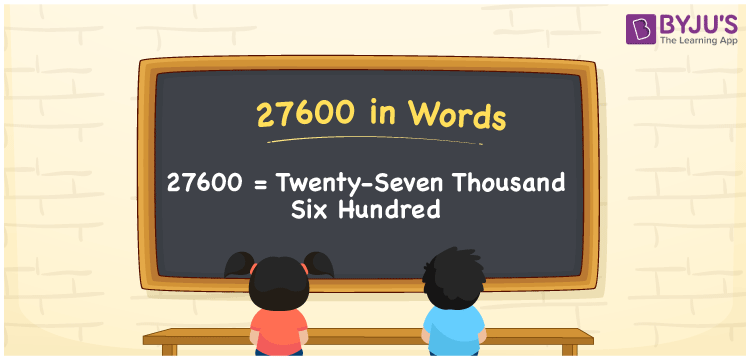# 27600 in Words

The number name of 27600 is twenty-seven thousand six hundred. We can say that the number 27600 is a cardinal number, as it helps to express the values. For example, there are about 27600 books in a library. For converting the number 27600 in words, the place value system is used. This article will guide you to write the number 27600 in words.

 27600 in Words: Twenty-seven Thousand Six Hundred. Twenty-seven Thousand Six Hundred in Numerical Form: 27600.

## 27600 in English Words## How to Write 27600 in Words?

The following place value table represents the five digit number 27600.

 Ten-thousands Thousands Hundreds Tens Ones 2 7 6 0 0

The expanded form of 27600 is as follows:

= 2 × Ten thousand + 7 × Thousand + 6 × Hundred + 0 × Ten + 0 × One

= 2 × 10000 + 7 × 1000 + 6 × 100 + 0 × 10 + 0 × 1

= 20000 + 7000 + 600

= 27600

= twenty-seven thousand six hundred

Hence, 27600 in words is twenty-seven thousand six hundred.

27600 in words – Twenty-seven thousand six hundred

Is 27600 an odd number? – No

Is 27600 an even number? – Yes

Is 27600 a perfect square number? – No

Is 27600 a perfect cube number? – No

Is 27600 a prime number? – No

Is 27600 a composite number? – Yes

## Frequently Asked Questions on 27600 in Words

Q1

### Write 27600 in words.

27600 in words is twenty-seven thousand six hundred.

Q2

### Simplify 27000 + 600, and express it in words.

Simplifying 27000 + 600, we get 27600. Hence, 27600 in words is twenty-seven thousand six hundred.

Q3

### Is 27600 a composite number?

Yes, 27600 is a composite number.